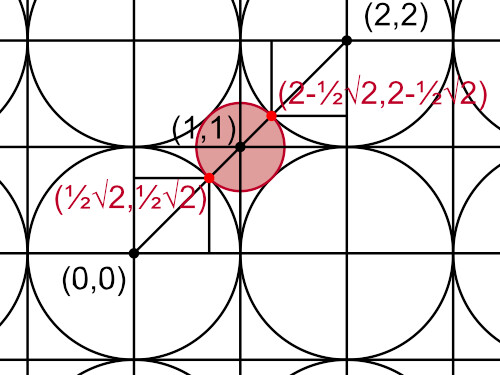Archive | 4:11 pm

## Square grid discs

10 Nov

Suppose we have a collection of discs with equal radius, neatly packed as a square grid. Between the discs there will be a space, where a smaller disc will fit in. What would be the radius of that smaller disc, relative to the radius of the larger discs?To simplify matters, suppose the large discs have a radius equal to 1. If we draw a line between one discs, say, with its center at (0,0), and a sphere that is to its right top, so with its center at (2,2), we can see, this line is at a 45 degrees angle. The little disc that fits in between the large discs has two intersection points on this line, as can be seen from the diagram above.

The distance between those intersection points is equal to the diameter of the little disc, or twice the radius of the little disc.According to Pythagoras’ theorem, the coordinates of one of the intersection points is (½√2,½√2).According to our diagram, the other intersection point is (2-½√2,2-½√2).

The diameter d of the little disc can be calculated as follows:

d² = (x2 – x1)² + (y2 – y1)²

Using the coordinate of the two intersection points, we get:

d² = ((2-½√2) – (½√2))² + ((2-½√2) – (½√2))²

which can be rewritten as:

d² = 2 × (2 – √2)²
d² = 2 × (4 + 2 – 4√2)
d² = 12 – 8√2
d = 2 × √(3 – 2√2)
r = d ÷ 2
r = √(3 – 2√2)

So, the radius of the little disc is equal to √(3 – 2√2). This means you have to multiply the radius of the large disc by this factor of √(3 – 2√2).

That is all.